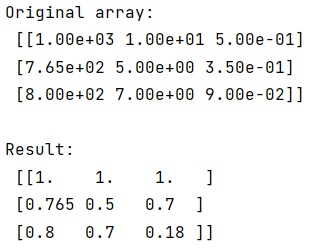# Normalize NumPy Array Columns

Learn how to normalize numpy array columns in Python?
Submitted by Pranit Sharma, on February 09, 2023

NumPy is an abbreviated form of Numerical Python. It is used for different types of scientific operations in python. Numpy is a vast library in python which is used for almost every kind of scientific or mathematical operation. It is itself an array which is a collection of various methods and functions for processing the arrays.

## NumPy Array - Normalizing Columns

Suppose we are a numpy array where each cell of a specific row represents a value for a feature. We need to normalize each column in such a way that each value lies between 0 and 1.

For this purpose, we will divide all the elements of the numpy array with the maximum of their respective row.

The arr.max() takes the maximum over the 0th dimension (i.e. rows). This gives us a vector of size (ncols,) containing the maximum value in each column. we will then divide x by this vector in order to normalize our values such that the maximum value in each column will be scaled to 1.

Let us understand with the help of an example,

## Python code to normalize numpy array columns

```# Import numpy
import numpy as np

# Creating a numpy array
arr =  np.array([[1000,  10,   0.5],
[ 765,   5,  0.35],
[ 800,   7,  0.09]])

# Display original array
print("Original array:\n",arr,"\n")

# Normalizing each column
res = arr/ arr.max(axis=0)

# Display Result
print("Result:\n",res,"\n")
```

Output: